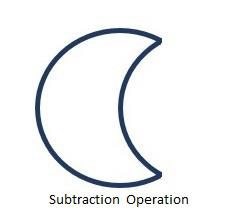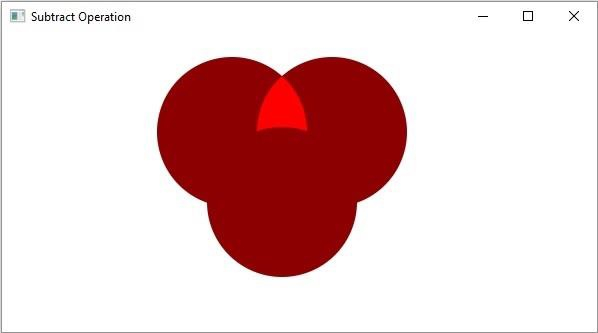# Explain the Subtract operation on 2D shapes in JavaFX

This operation takes two or more shapes as an input. Then, it returns the area of the first shape excluding the area overlapped by the second one as shown below.The subtract() (static) method of the javafx.scene.shape.Shape class accepts two Shape objects and returns the result of the subtract operation of the given objects.

## Example

import javafx.application.Application;
import javafx.scene.Group;
import javafx.scene.Scene;
import javafx.scene.paint.Color;
import javafx.stage.Stage;
import javafx.scene.shape.Circle;
import javafx.scene.shape.Shape;
public class JavaFXSubtractExample extends Application {
public void start(Stage stage) {
//Drawing circle1
Circle circle1 = new Circle();
circle1.setCenterX(230.0f);
circle1.setCenterY(100.0f);
circle1.setFill(Color.DARKRED);
//Drawing Circle2
Circle circle2 = new Circle();
circle2.setCenterX(280.0f);
circle2.setCenterY(170.0f);
circle2.setFill(Color.DARKRED);
//Drawing Circle3
Circle circle3 = new Circle();
circle3.setCenterX(330.0f);
circle3.setCenterY(100.0f);
circle3.setFill(Color.DARKRED);
//Intersect Operation
Shape subtract = Shape.subtract(circle1, circle2);
subtract = Shape.intersect(subtract, circle3);
subtract.setFill(Color.RED);
//Setting the stage
Group root = new Group(circle1, circle2, circle3, subtract);
Scene scene = new Scene(root, 595, 300);
stage.setTitle("Subtract Operation");
stage.setScene(scene);
stage.show();
}
public static void main(String args[]){
launch(args);
}
}

## Output